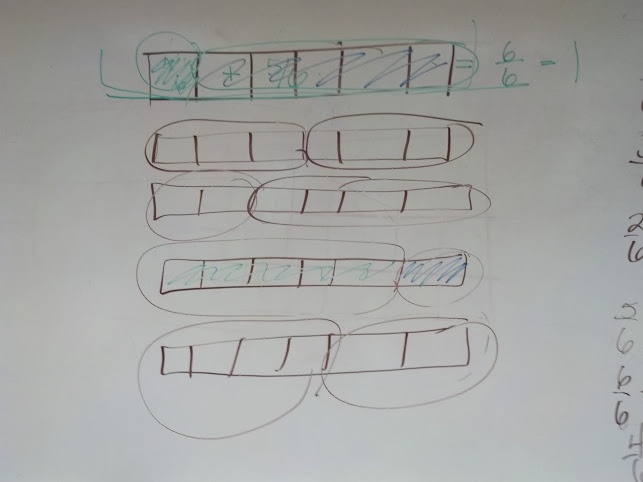# Fraction Partners of One Whole

1 teachers like this lesson
Print Lesson

## Objective

SWBAT find pairs of like fractions with the sum of 1.

#### Big Idea

Fraction Equivalence

## Number Hook

5 minutes

I played this interactive gopher video for my students again because yesterday they were so quiet when they first saw it. They had much more to say today.

This video works because of a mathematical trick with casting out nines.  My students did figure out that every number they ended up with was a multiple of nine.  I asked students if they will "always" get a multiple of nine when they follow the steps in the video and they are still unsure.  They need some more time to investigate this concept, but it is true that when you add up the sum of the digits in a number and subtract the single digit from your original number, you will always get a multiple of 9.

For example, lets say I picked a large number like 56879. 5 + 6 + 8 + 7 + 9= 35  3+5=8  When I subtract 8 from 56879 I get 56871.  56871 divided by 9 is 6319.

This trick only uses two number so the gopher only needs a chart to 100, but the same principle applies.

## Warm Up

12 minutes

I begin this warm up by reviewing the concept and definition of numerator and denominator by asking students to write down their full name in their math notebooks. Students write their first, middle, and last name. I then ask students to record what fraction of their name is vowels.  Most students do not have trouble doing this.   They know they need to find out how many letters are in their whole name and then record that as the denominator. Then they count the number of vowel letters and write that number as their numerator.

I ask several students to show their names and fractions under the document camera for the entire class to see.  As students show their fractions, I stress the words "whole, denominator and numerator."  Students are able to connect with the idea that the denominator is the number of letters in their whole name. I repeated things like:

So you're fraction is 9/22 because you have 9 vowels in your name and you have 22 letters that make up your whole name. So, 9 is your numerator because that's how many letters or parts are vowels, and 22 is the denominator because that's how many letters make up your whole name.

Next, I ask students to write down the fraction of their name that are not vowels. Again, the procedure is very similar to above except students share their fractions for the fraction of their name that is consonants.  As students display their fractions, I guide their thinking by asking them if they notice anything about the two fractions.  If a students is displaying 7/15 for the fraction of their name that is vowels and 8/15 for the fraction of their name that is consonants, I might say something like, "Do you notice anything about these two fractions?  What does 7 + 8 equal?"  Students very quickly say things like, "Yes, they add up to ALL of the letters!"

This then leads to the days lesson goal about finding partners of one.

## Concept Development

40 minutes

I begin this lesson by drawing a fraction bar on  the board. Students have had introductions to paper fraction strips and pattern blocks as tools to model fractions. This lesson incorporates fraction bars as another tool to help students visualize fraction regions.  I divide the fraction bar on the board into fifths and ask students what unit fraction I'm modeling.  Students respond with one fifth.

I tell students that this lesson's goal is to add fractions by finding partners of one.  For this lesson and unit, fraction partners of one refer to two fractions having like denominators and total a sum of one.  I remind students that  in first grade they discovered number partners for ten and tell them that today they will discover partners of one with different sized parts.

With the fraction bar drawn on the board, I circle one of the parts and ask students to identify what fraction of the whole bar I'm modeling. Students respond with 1/5 (one fifth). I then circle the other four parts and ask students the same question.  I then write 1/5 + 4/5 = _____.  I ask students what number they think should go in the blank. Students respond with 5/5 (five fifths) or 1.  From third grade experience, most all of my students know that when a fraction has the same numerator and denominator it is another name for 1.

As I write the addition equations for all partners of one with fifths, students also write the partners and model in their notebooks.  I then repeat this procedure with sixths.  See below for a photo of what this looks like. When I do sixths, I let students lists the partners. You can see below we started with 1/6 + 5/6 and then a student listed 3/6 + 3/6.  I talk with my students about how they would know whey they found all the partners of one. This is usually when a student or students discover the pattern of going in order by first circling one part, two parts, then three, etc.Then I assign students independent practice to find all the partners of one for 4/4, 8/8, and 7/7.  If students do not finish, they take this assignment home as homework.